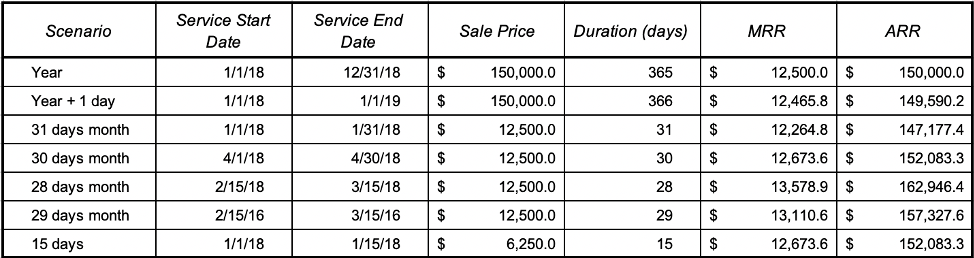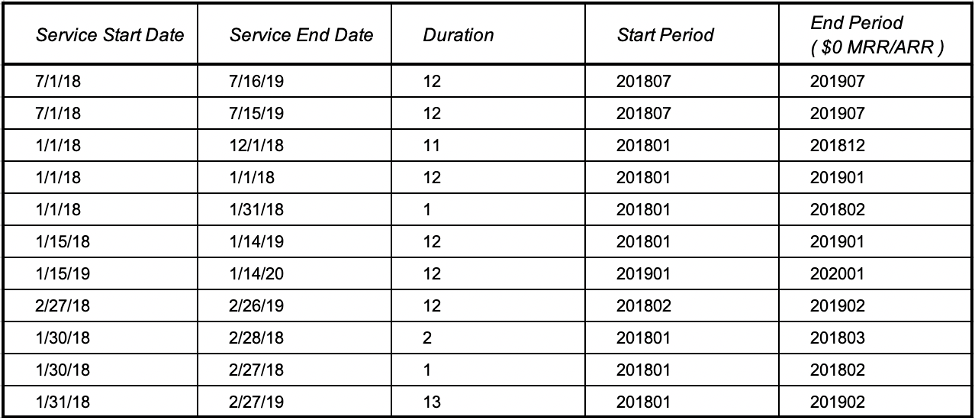# How are MRR and ARR calculated in RevRec?

Modified on: Thu, 5 Jan, 2023 at 9:44 PM

Scope

What is the formula used to calculate MRR and ARR in RevRec?

Types of examples of MRR and ARR calculation in RevRec?

Solution

RevRec calculates MRR using the following formula.

`MRR = ([Sale Price] / [Duration (days)]) * (365 / 12)ARR = ([Sale Price] / [Duration (days)]) * 365`

First, find the [Duration (days)] using [Service Start Date] and [Service End Date].

Calculate the [Daily Selling Price] for the subscription/recurring Item by dividing [Sale Price] by [Duration (days)]

MRR = Daily Selling Price x 365 /12

ARR = Daily Selling Price x 365

See below for some sample calculations that explain different scenarios.MRR/ARR Active Periods

MRR/ARR active period is established using [Service Start Date] & [Service End Date]. Although it is a pretty straightforward calculation there are some edge cases that need a closer look. Below we have tried to show several examples (including simple and edge cases) so that our users know exactly how active periods are established.Related Articles and Documentation

RevRec Standard Reports

Did you find it helpful? Yes No

Send feedback